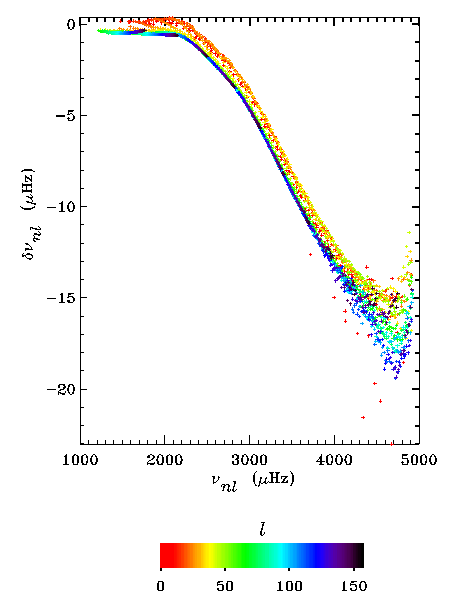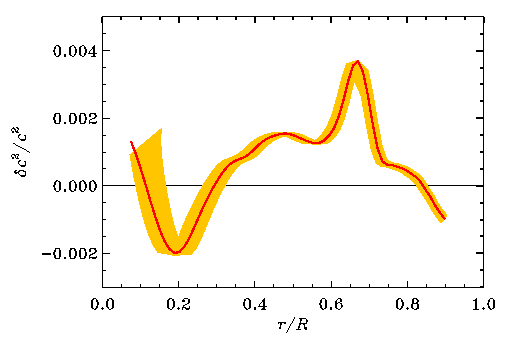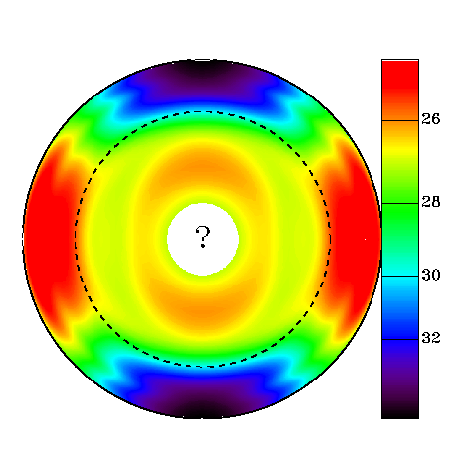# Helio- and Asteroseismology

INTRODUCTION  | OBSERVATIONS : the SUN  | OBSERVATIONS : STARS  | MODELS  | SOLAR OSCILLATIONS  | RESULTS
WHO ARE WE ?

## Results

After all of the hard work of observing for months and then converting the time sequence of images (movies) of the solar surface into a set of measured frequencies as a function of n, l and m, we are ready to compare and understand the differences between the measurements and our solar models, and use that to determine the conditions in the solar interior.

### Frequency differences

First we look at the differences between the frequencies of the Sun and those of the model :The figure is based on frequencies obtained by the GONG project.

Here the frequencies are shown in microherz, i.e. millionths of Hz ; an oscillation period of 5 minutes corresponds to a frequency of about 3000 microherz. The horizontal axis shows the observed oscillation frequency, and the vertical axis the difference between the Sun and the model frequencies, as (Sun)-(Model). Every point corresponds to an oscillation with a particular l and n. To differentiate between different oscillations the points are colour coded by l-value, as is shown on the scale below the figure.

The first thing that one can notice is that the differences are small : below 1 %. The model is therefore not very far from reality. Apart from that it is clear that the differences depend primarily on the frequency. That tells us that the cause of these differences must be very close to the surface of the Sun, where we know we have problems with modelling the convection. There are also differences between frequencies for oscillations with different l. That shows that part of the difference depends on how deeply the modes penetrate into the Sun, and therefore it shows that there are still errors deeper inside our model Sun.

(A small technical note: To bring out the effect more clearly, the frequency differences have been scaled ; this suppresses the effect of the varying depth of penetration of the different modes. The scale factor is 1 for radial modes, of degree 0, and decreases with increasing degree.)

### Differences in sound speed

We can use inversion methods to determine the difference between the sound speed of the Sun and that of our model, from the differences in the frequencies.The figure is based on frequencies obtained from the LOWL instrument.

The figure shows the difference in the square of the sound speed. The horizontal axis shows the distance to the centre of the Sun, in units of the solar radius. The yellow band gives a measure of the uncertainty in the determination due to the fact that we cannot determine the frequencies infinitely accurately : there are always measurement errors. Even so, we clearly see that we can determine the sound-speed difference almost everywhere in the Sun very precisely.

What have we learnt from this ? First of all, that our model of the Sun is very similar to the real thing : the difference between the two is less than 1/2 %. Since the square of the sound speed is more or less proportional to the temperature, the temperature inside the model is probably correspondingly close to reality. We have therefore succeeded in constructing the internal structure of the Sun quite precisely with numerical calculations, starting from our knowledge of physical laws.

On the other hand, the difference between the Sun and our model is quite a bit bigger than the errors coming from our measurements, so even if the model is very similar to the Sun it clearly is not correct. Therefore something is still wrong with the calculations. We are working very hard to understand what that "something" is.

### Rotation

We can also use the measured frequencies to infer the internal rotation rate of the Sun. Here there is no model that we can compare with : we have only a very poor understanding of all the processes that determine the rotation in the solar interior. The results of the seismic measurements are all the more interesting :The figure is based on frequency splittings obtained from the LOWL instrument.

The figure shows rotation in a cross-section of the Sun. The colour coding shows the rotation period in days, as indicated on the scale to the right. A good sign is that we find a surface rotation rate which is very similar to the one that we already knew from observations of features on the solar surface. The figure shows that the rotation rate varies with latitude in much the same way as on the surface, down to the dotted line. Inside this dotted line the rotation varies only very little.

The dotted line marks the boundary of the convection zone, where energy is transported through gas motion. The entire convection zone therefore rotates in much the same way that the surface does. The transition at the boundary of the convective zone, to constant rotation, seems to be quite sharp. However the details of this transition, and the cause of the variation of the rotation rate, are areas that are still the subject of very active research. The continued observations from GONG and SoHO will be of tremendous importance for this research. From the figure it is also clear that we don't actually know much about the rotation in the central parts of the Sun. This too we hope to learn much more about in the coming years, thanks to the new observations and also to the improved methods for analysing the results.